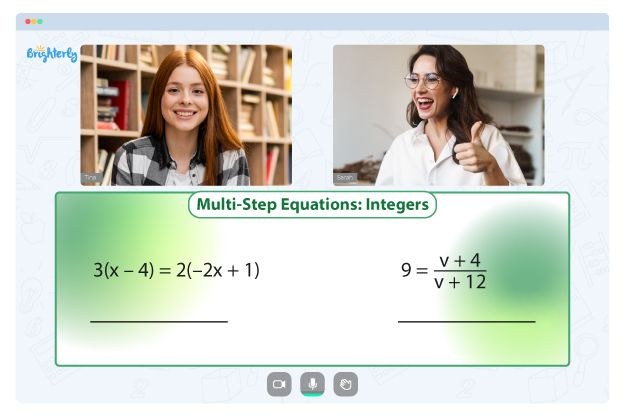# Factoring Worksheets

Factorization is the process of finding the prime factors of a number. Knowledge on how to factorize numbers is crucial for kids to understand other mathematics concepts. Factoring numbers is helpful in several mathematical disciplines, including algebra and geometry. Brighterly’s factoring worksheet with answers is an excellent resource to speed up your child’s understanding of this topic.

## Free factoring problems worksheet

Factoring entails expressing a number as the product of smaller numbers by reducing huge numbers to expressions in smaller numbers that are easy to deal with. Factoring makes solving multi-step arithmetic problems simpler.

Worksheets that focus on factoring help students get a more thorough knowledge of the idea and set them up for success when encountering more challenging mathematical material later on. For 4th graders and higher grade students, these worksheets will boost their factoring skills and grasp of the numerous factoring methods they have encountered in primary grades.

The factoring worksheet with answers also comprises a variety of problems on the prime factors of numbers. By working through these exercises, students may perfect their skills using the factor tree approach to establish if a given number is a composite or prime number.

The average student’s imagination is stimulated as they try to solve the challenging yet visually pleasing problems in Brighterly’s factoring problems worksheet. The G.C.F. and L.C.M.-based challenges in these worksheets can help students learn how to employ factoring to solve situations in the real world. Also, students may quickly understand multi-step arithmetic problems thanks to the information provided in the factoring worksheets.

Math for Kids

Is Your Child Struggling With Math?
1:1 Online Math Tutoring## Benefits of factors worksheet

When tutors first introduce factors to kids, the topic is difficult for them to understand. Brighterly’s worksheets are the go-to tools to aid the transition from counting and basic mathematics to factors and equations. They make learning and mastering factors easier.

Learning with the algebra 2 factoring worksheet boosts the critical abilities of kids. The excellent mix of complex and easy questions, coupled with the engaging design of the worksheets, enhances kids’ logical reasoning. Also, as kids learn with these worksheets, their analytical skills will improve significantly.

Their thinking changes as kids proceed from the simplest to complex questions. These challenging questions allow kids to think outside the box. The worksheets also improve the problem-solving abilities of kids, which is crucial in everyday life.

## Printable factoring worksheet PDF### Factoring Worksheets PDF

Factoring Worksheet### Factoring Worksheets PDF

Factoring Practice Worksheet### Factoring Worksheets PDF

Factoring Problems Worksheet### Factoring Worksheets PDF

Factoring Worksheet PDF

You can download the printable version of factoring worksheets from Brighterly’s website. Kids can learn independently and repeatedly with these printable worksheets. With these materials, learning becomes more straightforward and accessible to kids.

Tutors can also use the printable factoring practice worksheet to keep their kids busy. Also, they can build competitiveness among students by dividing the class into groups and assigning the worksheets using performance and time spent as a yardstick.

### More Factoring Worksheets

Need help with Equations?• Does your child struggle to grasp equations?
• Try studying with an online tutor.

Is your child having difficulties with understanding equations? An online tutor could provide the necessary guidance.